# Precalculus : Algebraic Vectors and Parametric Equations

## Example Questions

### Example Question #2 : Find A Direction Vector When Given Two Points

Find the vector through the points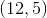and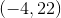.

Possible Answers: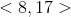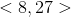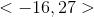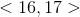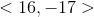Correct answer:Explanation:

The correct vector is given by the subtraction of the two points: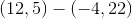.

Since the subtraction here is component-wise, it is given by the formula: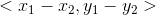.

This results in the vector.

The vector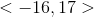is also correct as it is a scalar multiple of the vector marked as correct, it is found by reversing the order of the subtraction of the two points.

### Example Question #3 : Find A Direction Vector When Given Two Points

Find the vector that has the initial point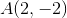and the terminal point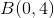.

Possible Answers: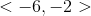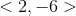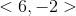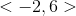Correct answer:Explanation:

To find the directional vector, subtract the coordinates of the initial point from the coordinates of the terminal point.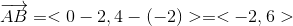### Example Question #4 : Find A Direction Vector When Given Two Points

Find the direction vector with an initial point of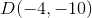and a terminal point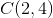.

Possible Answers: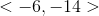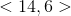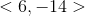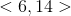Correct answer:Explanation:

To find the directional vector, subtract the coordinates of the initial point from the coordinates of the terminal point.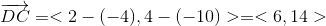### Example Question #1 : Find A Direction Vector When Given Two Points

Find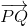if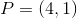and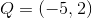.

Possible Answers: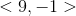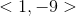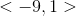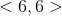Correct answer:Explanation:

To find the direction vector going fromto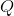, subtract the x and y-coordinates offrom.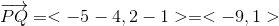### Example Question #6 : Find A Direction Vector When Given Two Points

Find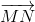if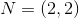and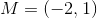.

Possible Answers: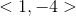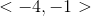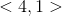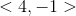Correct answer:Explanation:

To find the direction vector fromto, subtract the x- and y-coordinates offrom.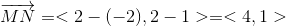### Example Question #7 : Find A Direction Vector When Given Two Points

Find the direction vector that has an initial point at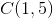and a terminal point of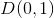.

Possible Answers: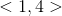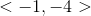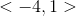Correct answer:Explanation:

To find the directional vector, subtract the coordinates of the initial point from the coordinates of the terminal point.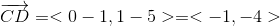### Example Question #8 : Find A Direction Vector When Given Two Points

Find the direction vector that has an initial point at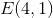and a terminal point at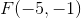.

Possible Answers: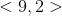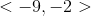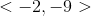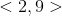Correct answer:Explanation:

To find the directional vector, subtract the coordinates of the initial point from the coordinates of the terminal point.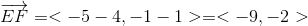### Example Question #9 : Find A Direction Vector When Given Two Points

Find the direction vector with an initial point of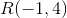and an terminal point of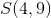.

Possible Answers: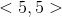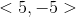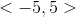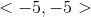Correct answer:Explanation:

To find the directional vector, subtract the coordinates of the initial point from the coordinates of the terminal point.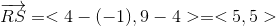### Example Question #10 : Find A Direction Vector When Given Two Points

Find the direction vector that has an initial point at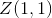and a terminal point at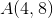.

Possible Answers: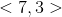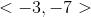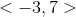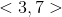Correct answer:Explanation:

To find the directional vector, subtract the coordinates of the initial point from the coordinates of the terminal point.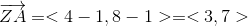### Example Question #11 : Algebraic Vectors And Parametric Equations

Find the direction vector with an initial point of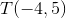and a terminal point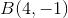.

Possible Answers: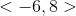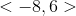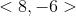Correct answer:Explanation:

To find the directional vector, subtract the coordinates of the initial point from the coordinates of the terminal point.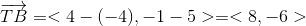### All Precalculus Resources